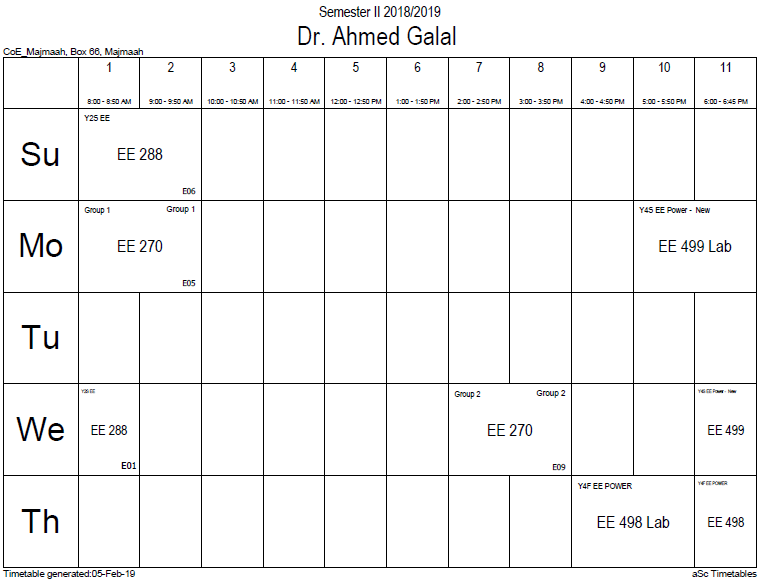## Circuit Laws

Magnetic circuits obey other laws that are similar to electrical circuit laws. For example, the total reluctance$scriptstyle mathcal{R}_T$ of reluctances$scriptstyle mathcal{R}_1, mathcal{R}_2, dots$ in series is:$R_T = R_1 + R_2 + cdots ,$

This also follows from Ampère's law and is analogous to Kirchhoff's voltage law for adding resistances in series. Also, the sum of magnetic fluxes$scriptstyle Phi_1, Phi_2, dots$ into any node is always zero:$Phi_1 + Phi_2 + cdots = 0. ,$

This follows from Gauss's law and is analogous to Kirchhoff's current law for analyzing electrical circuits.

Together, the three laws above form a complete system for analysing magnetic circuits, in a manner similar to electric circuits. Comparing the two types of circuits shows that:

• The equivalent to resistance R is the reluctance Rm
• The equivalent to current I is the magnetic flux Φ
• The equivalent to voltage V is the magnetomotive Force F

Magnetic circuits can be solved for the flux in each branch by application of the magnetic equivalent of Kirchhoff's Voltage Law (KVL) for pure source/resistance circuits. Specifically, whereas KVL states that the voltage excitation applied to a loop is equal to the sum of the voltage drops (resistance times current) around the loop, the magnetic analogue states that the magnetomotive force (achieved from ampere-turn excitation) is equal to the sum of MMF drops (product of flux and reluctance) across the rest of the loop. (If there are multiple loops, the current in each branch can be solved through a matrix equation—much as a matrix solution for mesh circuit branch currents is obtained in loop analysis—after which the individual branch currents are obtained by adding and/or subtracting the constituent loop currents as indicated by the adopted sign convention and loop orientations.) Per Ampère's law, the excitation is the product of the current and the number of complete loops made and is measured in ampere-turns. Stated more generally:$F = N,I = oint vec{H} cdot dvec{l}.$

(Note that, per Stokes's theorem, the closed line integral of H dot dl around a contour is equal to the open surface integral of curl H dot dA across the surface bounded by the closed contour. Since, from Maxwell's equations, curl H = J, the closed line integral of H dot dl evaluates to the total current passing through the surface. This is equal to the excitation, NI, which also measures current passing through the surface, thereby verifying that the net current flow through a surface is zero ampere-turns in a closed system that conserves energy.)

More complex magnetic systems, where the flux is not confined to a simple loop, must be analysed from first principles by using Maxwell's equations.

### Office HoursNo office hours### My Timetable### Contactsemail: [email protected]

Phone: 2570

### Welcome

Welcome To Faculty of Engineering### IEEEhttp://www.ieee.org/

/

### Bookmarkshttp://www.utk.edu/research/

http://science.doe.gov/grants/index.asp

http://www1.eere.energy.gov/vehiclesandfuels/

http://www.eere.energy.gov/

### Upcoming Conferences### Engineering quotes### Travel Web Siteshttp://www.hotels.com/

http://www.orbitz.com/

http://www.hotwire.com/us/index.jsp

http://www.kayak.com/

### Blackboardستقام اختبارات الميدتيرم يوم الثلاثاء 26-6-1440

حسب الجدول المعلن بلوحات الاعلان

### Summer trainingThe registration for summer training will start from 5th week of second semesterClass registration week 1

### برنامج التجسير### إحصائية الموقع

عدد الصفحات: 2879

البحوث والمحاضرات: 1280

الزيارات: 100942